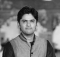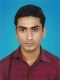## Symmetric Key AlgorithmsUpdated on 10-Jan-2023 10:36:58
What is Symmetric Key Algorithms? Symmetric key algorithms are a type of cryptographic technique that uses a shared secret key for both encryption and decryption. This means that the same key is used to encode and decode the message. Symmetric key algorithms are generally faster and more efficient than asymmetric key algorithms, but they require that the sender and receiver of a message share a secret key. Here are some of the basic principles of symmetric key algorithms − The same key is used for both encryption and decryption − In symmetric key algorithms, the same key is used ... Read More

## Simultaneous Localization and MappingUpdated on 30-Dec-2022 12:02:48
Introduction Simultaneous Localization and Mapping or SLAM is a method that let us build a map and locate our vehicles on that map at the same time. SLAM algorithms are used for unknown environment mapping and simultaneous localization. How is SLAM useful? Engineers can use SLAM for avoiding obstacles and also use them for path planning. SLAM software allows robot systems, drones, or autonomous vehicles to find paths in unknown environments and difficult terrains. This process involves a high amount of computing and processing power. SLAM can be useful for mapping areas that are too small or dangerous for ... Read More

## How to Calculate Percentiles For Monitoring Data?Updated on 30-Dec-2022 12:32:47
Introduction Monitoring online systems, especially which are data intensive is extremely essential for a continuous health check, analyzing and detecting downtimes, and improving performance. The percentile−based method is a very efficient technique to gauge the behavior of such a system. Let's have a look at this method. A General Refresher What are percentiles and why are they useful? In statistics, the value which indicates that below which a certain group of observations falls is called a percentile or centile. For example, for a student, if he/she has scored 90 percentile marks, it means that 90% of the students have scored ... Read More

## Introduction to Big O Notation in Data StructureUpdated on 21-Dec-2022 17:56:23
Introduction One of the most essential mathematical notations in computer science for determining an algorithm's effectiveness is the Big O notation. The length of time, memory, other resources, as well as a change in input size required to run an algorithm can all be used to evaluate how effective it is. Data structure's Big O Notation provides information about an algorithm's performance under various conditions. In other words, it provides the worst-case complexity or upper-bound runtime of an algorithm. Big O Notation in Data Structure A change in input size can affect how well an algorithm performs. Asymptotic notations, such ... Read More

## Difference between YOLO and SSDUpdated on 14-Oct-2022 11:45:58
YOLO and SSD are real-time object detection systems that possess significant differences, that have been listed below − YOLO (You Only Look Once) YOLO uses a neural network to help with real-time object detection. It became popular due to its speed and accuracy. It is considered a regression problem, where the algorithm looks at the object/s only once. There are algorithms associated with YOLO that achieve 155 FPS (frames per second). Image is divided into a grid, and every grid calculates class probabilities and bounding box parameters to determine the object in its entirety. It is an open-source detection ... Read More

## Difference Between Huffman Coding and Shannon Fano CodingUpdated on 21-Dec-2022 10:15:34
The primary function of a communication system is to transfer a message signal from a sender to the receiver. In the process of data transfer, the signal used to transfer the data should have the necessary properties required for the transmission as per the transmission channel. Hence, there are some information coding techniques used to transform the message signal into a transferrable signal through the transmission channel. Two of such information coding schemes are Huffman Coding and Shannon Fano Coding. The most basic difference between Huffman Coding and Shannon Fano Coding is that the Huffman coding provides a variable ... Read More

## Difference Between Tree and GraphUpdated on 21-Dec-2022 11:15:15
Both Trees and Graphs are types of non−linear data structures. They are different from each other in the context of their types of connections and loop formation. That means, a tree structure is connected such that it can never have loops, whereas a graph structure follows a network model and may have loops. Read this article to find out more about Tress and Graphs and how they are different from each other. What is Tree? A Tree is a non−linear data structure that is used to represent hierarchy. It is a set of nodes that are joined together to ... Read More

## Difference between Deterministic and Non-deterministic AlgorithmsUpdated on 09-Jun-2020 07:47:07
In the context of programming, an Algorithm is a set of well-defined instructions in sequence to perform a particular task and achieve the desired output. Here we say set of defined instructions which means that somewhere user knows the outcome of those instructions if they get executed in the expected manner.On the basis of the knowledge about outcome of the instructions, there are two types of algorithms namely − Deterministic and Non-deterministic Algorithms. Following are the main differences between both of the algorithms −Sr. No.KeyDeterministic AlgorithmNon-deterministic Algorithm1DefinitionThe algorithms in which the result of every algorithm is uniquely defined are known ... Read More

## Difference between BFS and DFSUpdated on 26-Nov-2019 12:03:08
BFS and DFS are graph traversal algorithms.BFSBreadth First Search (BFS) algorithm traverses a graph in a breadthward motion and uses a queue to remember to get the next vertex to start a search when a dead end occurs in any iteration.DFSDepth First Search (DFS) algorithm traverses a graph in a depthward motion and uses a stack to remember to get the next vertex to start a search when a dead end occurs in any iteration.Following are the important differences between BFS and DFS.Sr. No.KeyBFSDFS1DefinitionBFS, stands for Breadth First Search.DFS, stands for Depth First Search.2Data structureBFS uses Queue to find the ... Read More

## Bernoulli Distribution in Data StructuresUpdated on 27-Aug-2019 12:33:29
The Bernoulli Distribution is a discrete distribution having two possible outcomes labeled by x = 0 and x = 1. The x = 1 is success, and x = 0 is failure. Success occurs with probability p, and failure occurs with probability q as q = 1 – p. So$$P\lgroup x\rgroup=\begin{cases}1-p\:for & x = 0\p\:for & x = 0\end{cases}$$This can also be written as −$$P\lgroup x\rgroup=p^{n}\lgroup1-p\rgroup^{1-n}$$Example Live Demo#include #include using namespace std; int main(){    const int nrolls=10000;    default_random_engine generator;    bernoulli_distribution distribution(0.7);    int count=0; // count number of trues    for (int i=0; i